## January 6, 2016

### Decorated Cospans

#### Posted by John BaezI recently blogged about a paper I wrote with Brendan Fong. It’s about electrical circuits made of ‘passive’ components, like resistors, inductors and capacitors. We showed these circuits are morphisms in a category. Moreover, there’s a functor sending each circuit to its ‘external behavior’: what it does, as seen by someone who can only measure voltages and currents at the terminals.

Our paper uses a formalism that Brendan developed here:

• Brendan Fong, Decorated cospans, Theory and Applications of Categories 30 (2015), 1096–1120.

Let me explain this formalism. You don’t need to be an electrical engineer to like this!

The idea here is we may want to take something like a graph with edges labelled by positive numbers: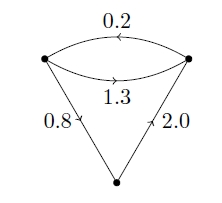and say that some of its nodes are ‘inputs’, while others are ‘outputs’: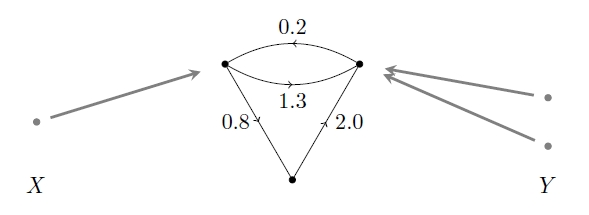This lets us treat our labelled graph as a ‘morphism’ from the set $X$ to the set $Y$.

The point is that we can compose such morphisms. For example, suppose we have another one of these things, going from $Y$ to $Z$: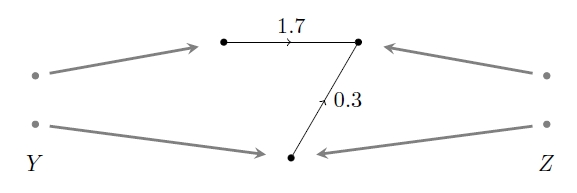Since the points of $Y$ are sitting in both things: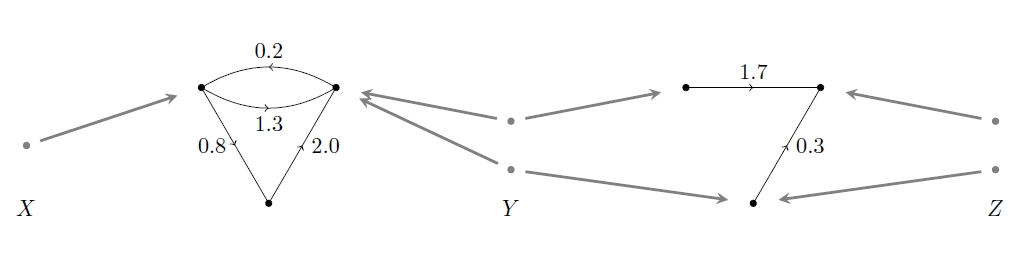we can glue them together and get a thing going from $X$ to $Z$: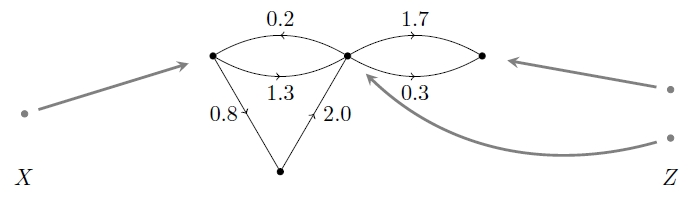That’s how we compose these morphisms.

Note how we’re specifying some nodes of our original thing as inputs and outputs:We’re using maps from two sets $X$ and $Y$ to the set $N$ of nodes of our graph. And a bit surprisingly, we’re not demanding that these maps be one-to-one. That turns out to be useful—and in general, when doing math, it’s dumb to make your definitions forbid certain possibilities unless you really need to.

So, our thing is really a cospan of finite sets—that is, a diagram of finite sets and functions like this: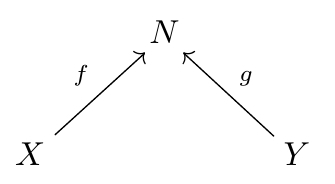together some extra structure on the set $N$. This extra structure is what Brendan calls a decoration, and it makes the cospan into a ‘decorated cospan’. In this particular example, a decoration on $N$ is a way of making it into the nodes of a graph with edges labelled by positive numbers. But one could consider many other kinds of decorations: this idea is very general.

To formalize the idea of ‘a kind of decoration’, Brendan uses a functor

$F: {FinSet} \to {Set}$

sending each finite set $N$ to a set of $F(N)$. This set $F(N)$ is the set of decorations of the given kind that we can put on $N$.

So, for any such functor $F$, a decorated cospan of finite sets is a cospan of finite sets:together with an element of $F(N)$.

But in fact, Brendan goes further. He’s not content to use a functor

$F: {FinSet} \to {Set}$

to decorate his cospans.

First, there’s no need to limit ourselves to cospans of finite sets: we can replace ${FinSet}$ with some other category! If $C$ is any category with finite colimits, there’s a category ${Cospan}(C)$ with:

• objects of $C$ as its objects,
• isomorphism classes of cospans between these as morphisms.

Second, there’s no need to limit ourselves to decorations that are elements of a set: we can replace ${Set}$ with some other category! If $D$ is any symmetric monoidal category, we can define an element of an object $d \in D$ to be a morphism

$f: I \to d$

where $I$ is the unit for the tensor product in $D$.

So, Brendan defines decorated cospans at this high level of generality, and shows that under some mild conditions we can compose them, just as in the pictures we saw earlier.

Here’s one of the theorems Brendan proves:

Theorem. Suppose $C$ is a category with finite colimits, and make $C$ into a symmetric monoidal category with its coproduct as the tensor product. Suppose $D$ is a symmetric monoidal category, and suppose $F: C \to D$ is a lax symmetric monoidal functor. Define an F-decorated cospan to be a cospanin $C$ together with an element of $F(N)$. Then there is a category with

• objects of $C$ as its objects,
• isomorphism classes of $F$-decorated cospans as its morphisms.

This is called the F-decorated cospan category, $FCospan$. This category becomes symmetric monoidal in a natural way. It is then a dagger compact category.

(You may not know all this jargon, but ‘lax symmetric monoidal’, for example, talks about how we can take decorations on two things and get a decoration on their disjoint union, or ‘coproduct’. We need to be able to do this—as should be obvious from the pictures I drew. Also, a ‘dagger compact category’ is the kind of category whose morphisms can be drawn as networks.)

Brendan also explains how to get functors between decorated cospan categories. We need this in our paper on electrical circuits, because we consider several categories where morphisms is a circuit, or something that captures some aspect of a circuit. Most of these categories are decorated cospan categories. We want to get functors between them. And often we can just use Brendan’s general results to get the job done! No fuss, no muss: all the hard work has been done ahead of time.

I expect to use this technology a lot in my work on network theory. You’ll see another application in my next post here!

Posted at January 6, 2016 12:39 PM UTC

TrackBack URL for this Entry:   https://golem.ph.utexas.edu/cgi-bin/MT-3.0/dxy-tb.fcgi/2859

### Re: Decorated Cospans

Since this is the $n$-Category Café, let me ask the obvious question: is there a bicategory of decorated cospans where you don’t have to quotient by isomorphism?

Posted by: Mike Shulman on January 8, 2016 4:24 AM | Permalink | Reply to this

### Re: Decorated Cospans

Good question! I have a student, Kenny Courser, working to prove that.

In this network theory project I want to go as far as I can with categories before bringing in higher categories: getting applied mathematicians, engineers or biologists to start using categories will be tough, but if they do, $n$-categories are sure to follow.

Posted by: John Baez on January 8, 2016 7:02 AM | Permalink | Reply to this

### Re: Decorated Cospans

Hello, interesting ideas.

When I talked with Brendan about this last summer, I had the impression that there is actually no extra generality by moving from $\mathbf{Set}$ to an arbitrary category $D$ (for the decorations).

I had the idea that the definition of “decorated cospan” remains the same if we compose the monoidal functor $F:C\to D$ with the global sections functor $D(I,-):D\to \mathbf{Set}$, which is always monoidal. So we may as well take $D=\mathbf{Set}$.

Am I wrong?

Posted by: Sam Staton on January 25, 2016 5:15 PM | Permalink | Reply to this

### Re: Decorated Cospans

That sounds plausible. Thanks for pointing that out! I’ll bring this to Brendan’s attention: he should mention this in his thesis, if it’s true.

It may also help Kenny Courser.

Posted by: John Baez on January 25, 2016 5:49 PM | Permalink | Reply to this

### Re: Decorated Cospans

You maybe interested in looking into the field of ‘port-Hamiltonian’ systems, which is very connected to systems you discuss in this post.

Posted by: pp on March 19, 2016 1:31 PM | Permalink | Reply to this

Post a New Comment